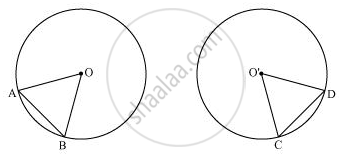# Prove that If Chords of Congruent Circles Subtend Equal Angles at Their Centres, Then the Chords Are Equal. - Mathematics

Prove that if chords of congruent circles subtend equal angles at their centres, then the chords are equal.

#### Solution

Let us consider two congruent circles (circles of same radius) with centres as O and O'.In ΔAOB and ΔCO'D,

∠AOB = ∠CO'D (Given)

OA = O'C (Radii of congruent circles)

OB = O'D (Radii of congruent circles)

∴ ΔAOB ≅ ΔCO'D (SAS congruence rule)

⇒ AB = CD (By CPCT)

Hence, if chords of congruent circles subtend equal angles at their centres, then the chords are equal.

Concept: Angle Subtended by a Chord at a Point
Is there an error in this question or solution?
Chapter 10: Circles - Exercise 10.2 [Page 173]

#### APPEARS IN

NCERT Class 9 Maths
Chapter 10 Circles
Exercise 10.2 | Q 2 | Page 173

Share